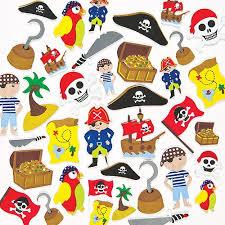# Two-thirds 3030

Peter is a 5th-grade student. He collects stickers. He has two-thirds of them in the album, a quarter is ready for exchange, and he wants to give eight stickers to his friends. How many stickers does Peter have?

x =  96

### Step-by-step explanation:

2/3 x + 1/4 x + 8 = x

2/3·x + 1/4·x + 8 = x

x = 96

x = 96/1 = 96

x = 96

Our simple equation calculator calculates it.Did you find an error or inaccuracy? Feel free to write us. Thank you!

Tips for related online calculators
Need help calculating sum, simplifying, or multiplying fractions? Try our fraction calculator.
Do you have a linear equation or system of equations and looking for its solution? Or do you have a quadratic equation?